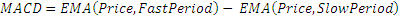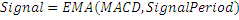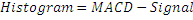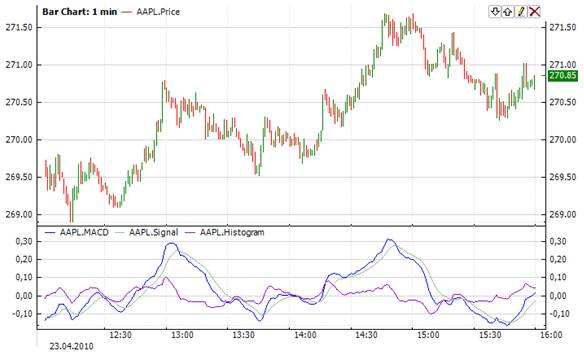﻿Moving Average Convergence

# Moving Average Convergence

The Moving Average Convergence/Divergence (Macd) is the difference between two Exponential Moving Averages(Ema). The Signal line is an Exponential Moving Average of the Macd.  The Macd signals trend changes and indicates the start of new trend direction. High values indicate overbought conditions, low values indicate oversold conditions. Divergence with the price indicates an end to the current trend, especially if the Macd is at extreme high or low values.Market Signals

When the Macd line crosses above the signal line a buy signal is generated. When the Macd crosses below the signal line a sell signal is generated. It is also popular to buy/sell when the Macd goes above/below zero.CalculationChart ExampleImplementation and Usage

To calculate Macd in a strategy you can use the following constructors:

Macd – sets default values:  fastPeriod = 12, slowPeriod = 26, signalPeriod = 9.

Macd(Int32, Int32, Int32) – sets periods for indicator

Use

MACD

Signal

Histogram

properties to get current value.Example
C#
``` 1// Create new instance
2Macd macd = new Macd(14, 28, 9);
3
4// Number of stored values
5macd.HistoryCapacity = 2;
6
9
10// Get indicator value
11double IndMACD = macd.MACD;
12double IndSignal = macd.Signal;
13double IndHistogram = macd.Histogram;
14
15// Get previous values
16double IndPrevMACD = macd.MACD;
17double IndPrevSignal = macd.Signal;
18double IndPrevHistogram = macd. Histogram;
19Checks for Signal line crosses MACD line:
20using FinAnalysis.TA;
21using FinAnalysis.Base;
22Macd macd;
23
24public override void OnInit()
25{
26    //...
27    // Create new instance, MACD(14, 28, 9)
28    macd = new Macd(14, 28, 9);
29
30    // Store 2 indicator values
31    macd.HistoryCapacity = 2;
32    //...
33}
34
35public override void OnBarClose()
36{
37    //...
39
40    // Checks for values in memory
41    if (macd.HistoryCount >= 2)
42    {
43        // Checks for cross
44        if (macd.MACD > macd.Signal && macd.MACD <= macd.Signal)
45        {
46            // Long position
47        }
48
49
50
51        if (macd.MACD < macd.Signal && macd.MACD >= macd.Signal)
52        {
53            // Short position
54        }
55    }
56    //...
57}```